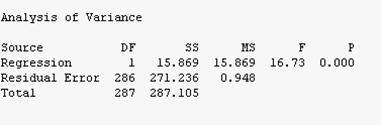# Minitab Help - Learn All the Steps Necessary to Run a Linear Regression Analysis with Minitab

• Minitab is a powerful Statistical software package with a very intuitive graphical interface. In Unit 3 you tested 2 different sets of hypotheses using the database from Unit 1. Provide a summary of your findings. Address each hypothesis you tested.
$\begin{array}{cc} & {{H}_{0}}:{{\mu }_{M}}={{\mu }_{F}} \\ & {{H}_{1}}:{{\mu }_{M}}\ne {{\mu }_{F}} \\ \end{array}$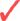Need Help with Minitab?We can help! Your satisfaction is guaranteed.Our rate starts at \$35/hour. Free quote in hours. Quick turnaround!

## Minitab T-test results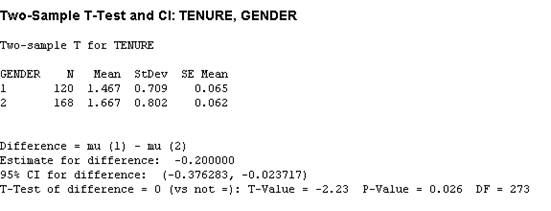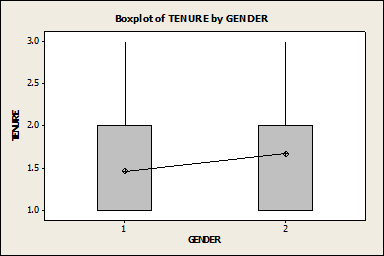$\begin{array}{cc} & {{H}_{0}}:{{\mu }_{I}}=5 \\ & {{H}_{1}}:{{\mu }_{I}}\ne 5 \\ \end{array}$

## One sample t-test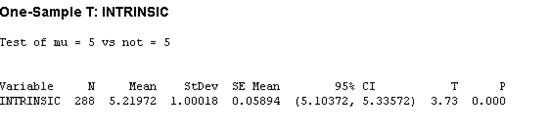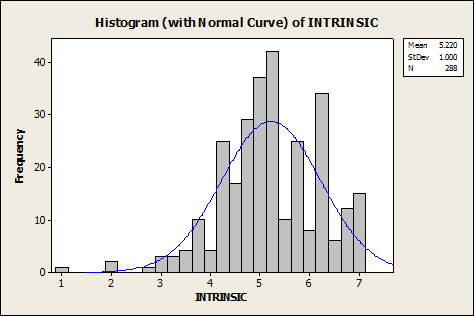• In Unit 4 you researched the topic on Job Satisfaction. Provide a one page summary of your research findings. Are your findings consistent with what you observed when you tested your hypotheses in the Unit 3 assignment?
• 57.3% (5,062) said the primary reason was because they believe they were underpaid
• Within this group, 1,624 employees provided details of their current job and salary
• Considering current market rates, only 18.7% were actually underpaid.
• Work through a simple regression calculation using intrinsic job satisfaction and extrinsic job satisfaction. You are more than welcome to select thirty data points from our Unit 1 data set to use for each group. You are also welcome to decide which group you want to make the dependent variable and which the independent variable. The final answer will then be a complete model equation resembling: Y = bx + a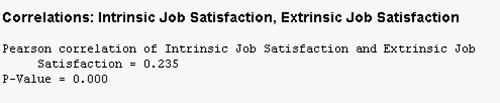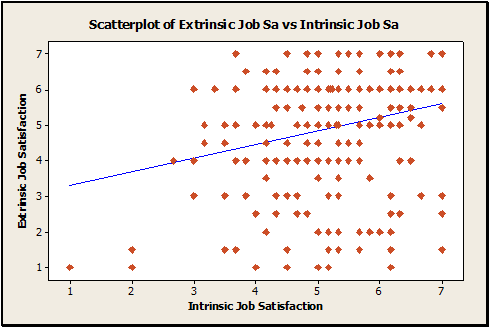weak.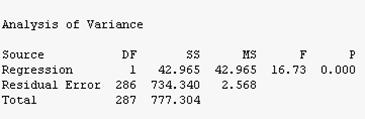### Linear Regression Results obtained with Minitab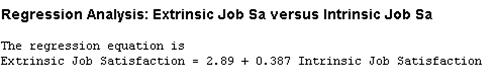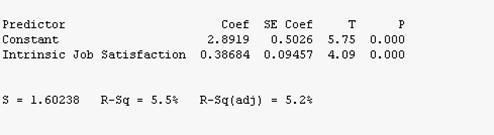$Extrinsic=2.89+0.387\text{ }Intrinsic$
• Work through a second simple regression by switching the dependent and independent variables used in the first regression. Make very specific comments and give reasons regarding any similarities or differences in Multiple R and the ANOVA coefficients.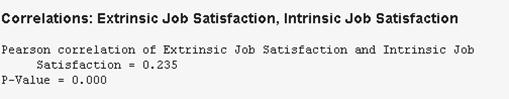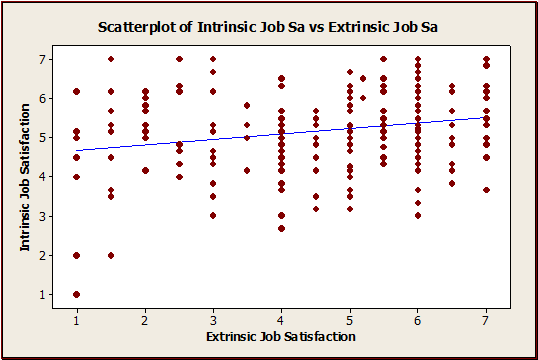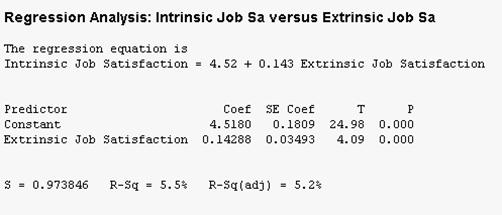$Intrinsic = 4.52 + 0.143\text{ }Extrinsic$